Author: Oscar Cronquist Article last updated on May 21, 2022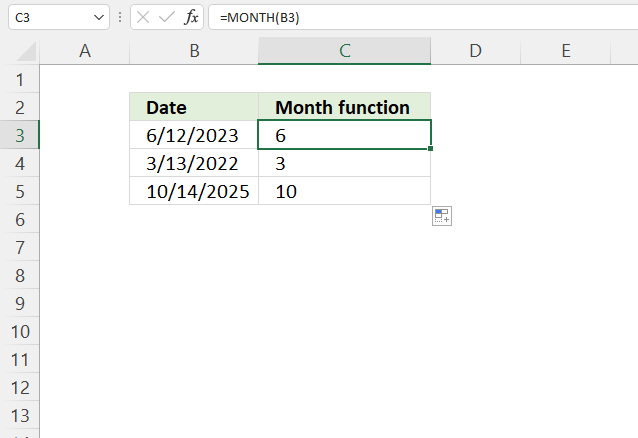The MONTH function extracts the month as a number from an Excel date.

• 1 - January
• 2 - February
• 3 - March
• 4 - April
• 5 - May
• 6 - June
• 7 - July
• 8 - August
• 9 - September
• 10 - October
• 11 - November
• 12 - December

## 1. MONTH Function Syntax

MONTH(serial_number)

## 2. MONTH Function Arguments

 serial_number Required. The date value you want to extract the month from.

## 3. MONTH Function not working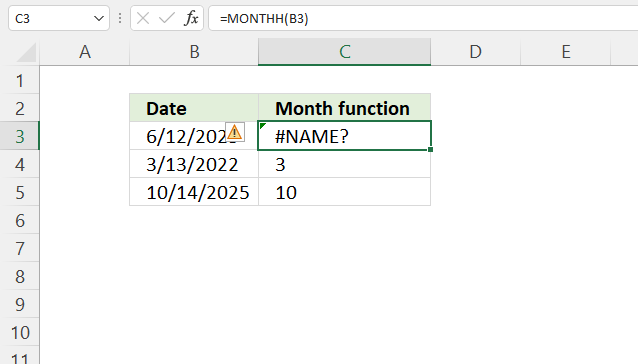Check your spelling, the image above shows the MONTH function misspelled. An #NAME? error is shown in cell C3.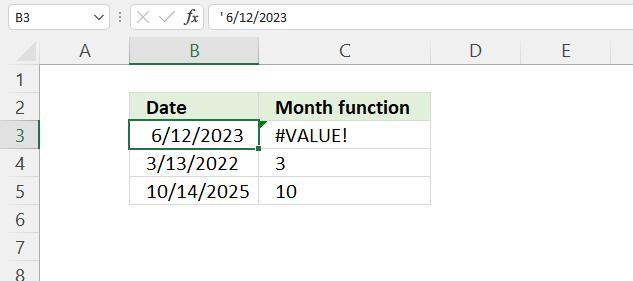Cell B3 contains a text value and not a proper Excel date, this returns a #VALUE! error in cell C3.

## 4. MONTH Function exampleFormula in cell C3:

=MONTH(B3)

To understand the MONTH function I need to explain that it needs an Excel date in order to calculate the month properly.  The date in cell B3 is an Excel date meaning it is a number formatted as a date. 1 is 1/1/1900 and 1/1/2000 is 36526 meaning there are 36526 days between the dates.

You can verify this, select a cell containing 1/1/2000 and press CTRL + 1 to open the "Format Cells" dialog box.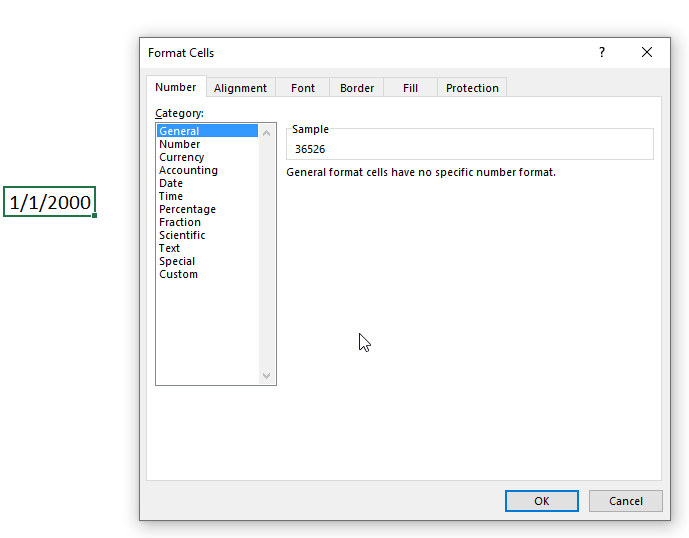The "Sample" shown in the image above demonstrates what the value changes to if you choose to format the cells using the category "General". The sample contains 36526 which is the number representing 1/1/2000.

## 5. MONTH Function alternative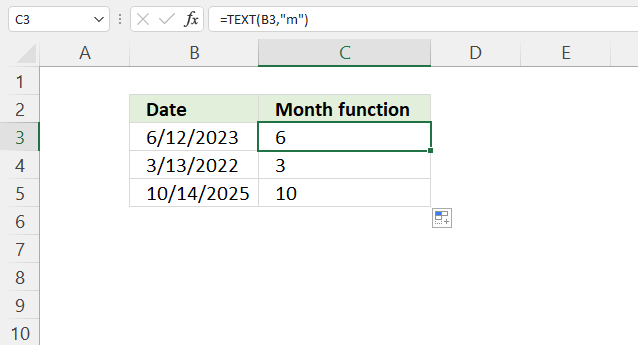Formula in cell C3:

=TEXT(B3, "m")

### Explaining formula

#### Step 1 - TEXT function

The TEXT function lets you format values.

TEXT(valueformat_text)

value - The string you want to format. You can use a cell reference here or use a text string.

format_text - Formatting code allowing you to change the way, for example, a date or a number is displayed to the Excel user.

#### Step 2 - Populate arguments

The TEXT function has two arguments.

value - B3

format_text - "m"

"m" is an abbreviation for month. A single "m" returns the number representing the position of a given month in a year.

#### Step 3 - Evaluate TEXT function

TEXT(B3, "m")

becomes

TEXT(45089, "m")

and returns 6. 6 represents the sixth month in a year which is "June".

## 6. MONTH function - return month name instead of a number

### 6.1 Example 1 - INDEX function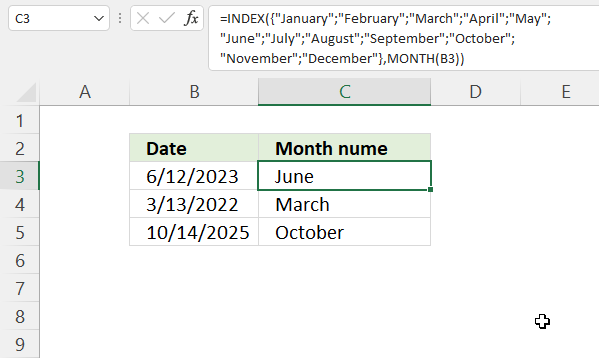Formula in cell C3:

=INDEX({"January"; "February"; "March"; "April"; "May"; "June"; "July"; "August"; "September"; "October"; "November"; "December"},MONTH(B3))

#### Explaining formula in cell C3

Step 1 - Calculate month number

MONTH(B3)

• 1 - January
• 2 - February
• 3 - March
• 4 - April
• 5 - May
• 6 - June
• 7 - July
• 8 - August
• 9 - September
• 10 - October
• 11 - November
• 12 - December

MONTH(B3)

becomes

MONTH(45089)

and returns 6. Number 6 represents "June".

Step 2 - Return corresponding month name

The INDEX function returns a value in a cell range or array based on a row and column number (optional).

INDEX(array[row_num][column_num], [area_num])

INDEX({"January"; "February"; "March"; "April"; "May"; "June"; "July"; "August"; "September"; "October"; "November"; "December"},MONTH(B3))

becomes

INDEX({"January"; "February"; "March"; "April"; "May"; "June"; "July"; "August"; "September"; "October"; "November"; "December"}, 6)

and returns "June". "June" is the sixth month in a year.

### 6.2 Example 2 - TEXT function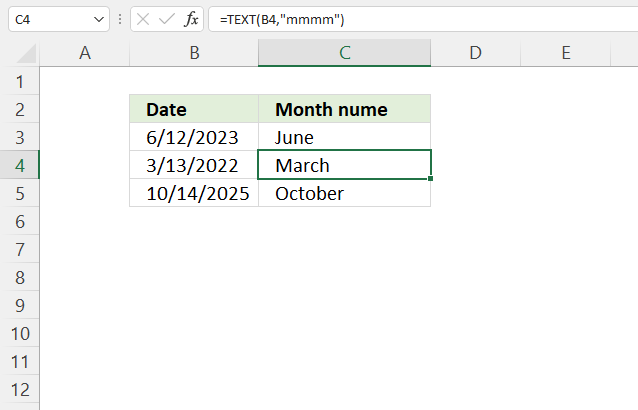Formula in cell C4:

=TEXT(B4, "mmmm")

#### Explaining formula in cell C4

The TEXT function lets you format values.

TEXT(valueformat_text)

value - The string you want to format. You can use a cell reference here or use a text string.

format_text - Formatting code allowing you to change the way, for example, a date or a number is displayed to the Excel user.

Formatting code "mmmm" evaluates to the month name.

TEXT(B4, "mmmm")

becomes

TEXT(44603, "mmmm")

and returns "March".

### 6.3 Example 3 - Cell formatting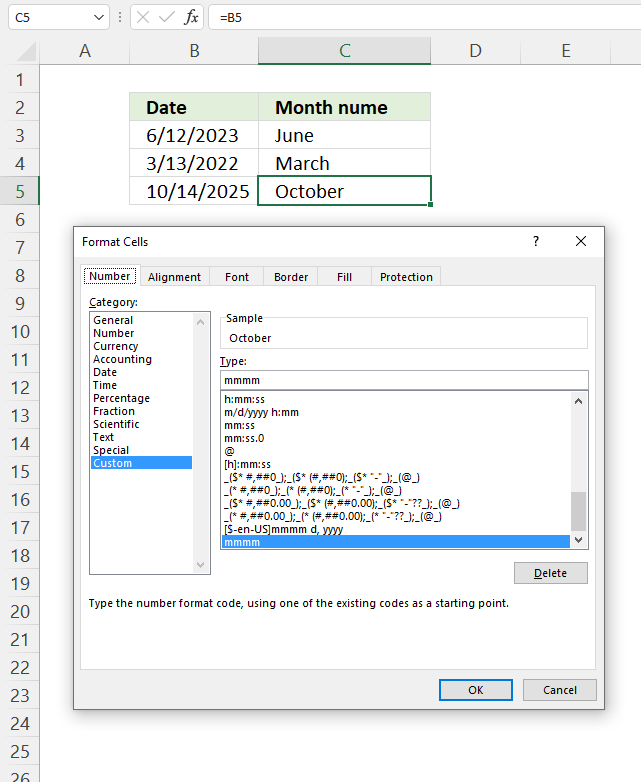You can also show the month name using cell formatting, the image above demonstrates cell formatting applied to cell C5.

How to apply cell formatting:

1. Select cell C5.
2. Press CTRL + 1 to open the "Format Cells" dialog box.
3. Press with left mouse button on "Category" Custom, see the image above.
4. Enter mmmm below Type:
5. Press with left mouse button on OK button to apply cahnges.

## 7. Filter dates based on month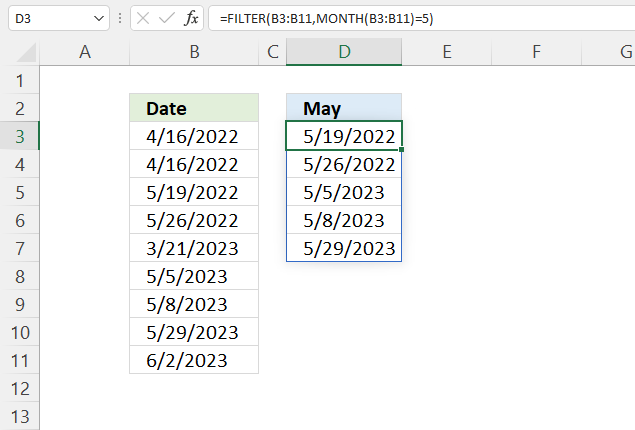Formula in cell D3:

=FILTER(B3:B11,MONTH(B3:B11)=5)

### Explaining formula

#### Step 1 - Calculate month number for each date value

MONTH(B3:B11)

becomes

MONTH({44667; 44667; 44700; 44707; 45006; 45051; 45054; 45075; 45079})

and returns {4; 4; 5; 5; 3; 5; 5; 5; 6}.

#### Step 2 - Compare month number to condition

The equal sign lets you check if values are equal, note that this does not perform a case-sensitive comparison. Check out the EXACT function if upper and lower letters matter.

MONTH(B3:B11)=5

becomes

{4; 4; 5; 5; 3; 5; 5; 5; 6}=5

and returns

{FALSE; FALSE; TRUE; TRUE; FALSE; TRUE; TRUE; TRUE; FALSE}

#### Step 3 - Extract dates meeting the condition

The FILTER function is a new function available to Excel 365 subscribers. It lets you extract values based on a condition or criteria.

FILTER(arrayinclude, [if_empty])

FILTER(B3:B11, MONTH(B3:B11)=5)

becomes

FILTER({44667; 44667; 44700; 44707; 45006; 45051; 45054; 45075; 45079}, {FALSE; FALSE; TRUE; TRUE; FALSE; TRUE; TRUE; TRUE; FALSE})

and returns {44700; 44707; 45051; 45054; 45075}.